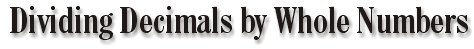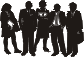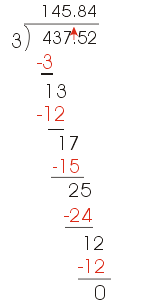###The Ohio State Park Service decided to split the current value of the bonds, \$16,438.84, among the descendants of Mr.Gray. Fourteen people were able to trace their relationship back to Mr. Gray.

To divide decimals by whole numbers, follow these steps:

• Rewrite the problem with a division box.
• Place the decimal point in the quotient
directly above the decimal point in the box.
• Ignore the decimal point and divide in the   same way you would divide whole numbers.

 If each of the 14 descendants received an equal share of the stocks' value, how much would each person get?Example: Place the decimal point directly above the decimal point in the box.Decimals Main | Go to Dividing Decimals by Decimals